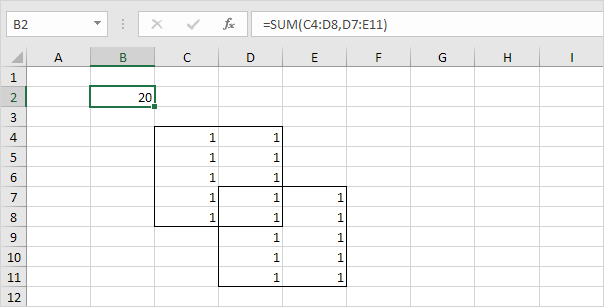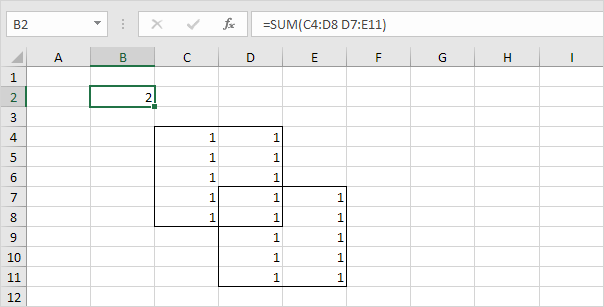# How To Use The Union And Intersect Operator In Excel

Jan 31, 2020 • edited Feb 01, 2020A couple of rarely used features of worksheet formulas are Unions and Intersects.

This example illustrates how to use the union and intersect operator (borders below for illustration only) in Excel.
1. The union operator (comma) adds two ranges.Explanation: the SUM function reduces to =SUM(C4:D8) + SUM(D7:E11), 20.

2. The intersect operator (single space) returns the intersection of two ranges.Explanation: the SUM function reduces to =SUM(D7:D8), 2.

#How To#Tutorial#Range#Union and Intersect

How To Use Flash Fill in Excel

How to Transpose Columns to Rows Use Paste Special in Excel